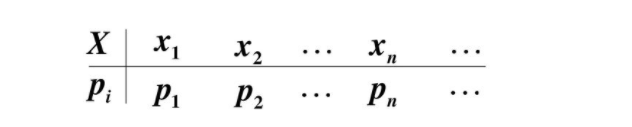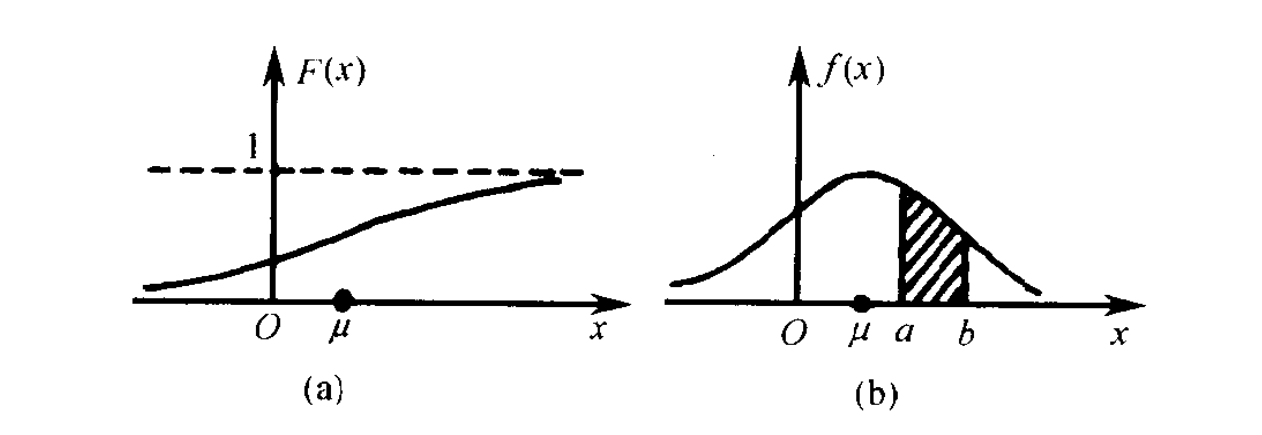# 《概率论与数理统计》之概率函数、概率分布函数与概率密度函数理解

### 离散型随机变量的概率函数与概率分布函数

X X 是离散型随机变量，其全部可能取值是 a 1 , a 2 , . . . , a n {a_{1},a_{2},...,a_{n}} ，则它的概率函数表示为：
p i = P ( X = a i ) ( i = 1 , 2 , . . . , n ) p_{i} = P(X = a_{i}) \quad (i=1,2,...,n)X X 为随机变量，则随机变量的概率分布函数为：
P ( X ≤ x ) = F ( x ) ( − ∞ &lt; x &lt; ∞ ) P(X \leq x) = F(x) \quad (- \infty &lt;x&lt; \infty)

F ( x ) = P ( X ≤ x ) = ∑ { i ∣ a i ≤ x } p i F(x) = P(X \leq x) = \sum_{\{i|a_{i}\leq x\}}p_{i}

### 连续型随机变量的概率密度函数与概率分布函数

1、 f ( x ) ≥ 0 f(x)\geq0
2、 ∫ − ∞ ∞ f ( x ) d x = 1 \int_{-\infty}^{\infty}f(x)dx = 1
3、对于任何常数 a &lt; b a&lt;b ，有 P ( a ≤ X ≤ b ) = F ( b ) − F ( a ) = ∫ a b f ( x ) d x P(a\leq X \leq b) = F(b) - F(a) = \int_{a}^{b}f(x)dx### 总结

1、离散型随机变量的概率函数和连续型随机变量的概率密度函数都用来描述随机变量如何在所有取值处分配总的概率1。离散型随机变量的概率函数可以直接得到离散型变量对应的概率值，连续型随机变量则是通过一个区间内概率密度函数的面积来表示概率值。
2、无论是离散型还是连续型概率分布函数，可以按照它的另一个叫法累积概率函数来理解，既然是累积概率，那么得到的公式是一个求和的形式，得到的图像是一个递增的过程。
3、由于概率分布的研究多是侧重于连续型随机变量，毕竟连续型随机变量的概率分布函数和概率密度函数作为连续函数，还有很多可以研究的点，所以后期会再整理一些常见的连续型随机变量的概率分布函数和概率密度函数。

### REF

《概率论与数理统计（陈希孺编著）》
https://www.jianshu.com/p/b570b1ba92bb

09-24465
12-11157
11-152685
03-221403
04-216万+
04-182498
05-294295
11-297482
03-212076
09-1111万+
03-261万+
11-03475
03-221万+
09-28331
10-011万+
02-19540
10-041068
09-28781
01-18402

### “相关推荐”对你有帮助么？

•非常没帮助
•没帮助
•一般
•有帮助
•非常有帮助被折叠的  条评论 为什么被折叠?到【灌水乐园】发言¥2 ¥4 ¥6 ¥10 ¥20余额支付 (余额：-- )扫码支付获取中扫码支付点击重新获取扫码支付1.余额是钱包充值的虚拟货币，按照1:1的比例进行支付金额的抵扣。
2.余额无法直接购买下载，可以购买VIP、C币套餐、付费专栏及课程。余额充值